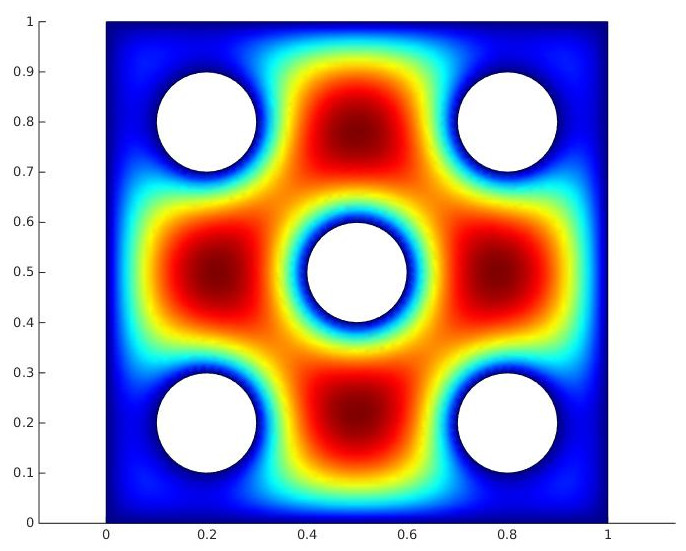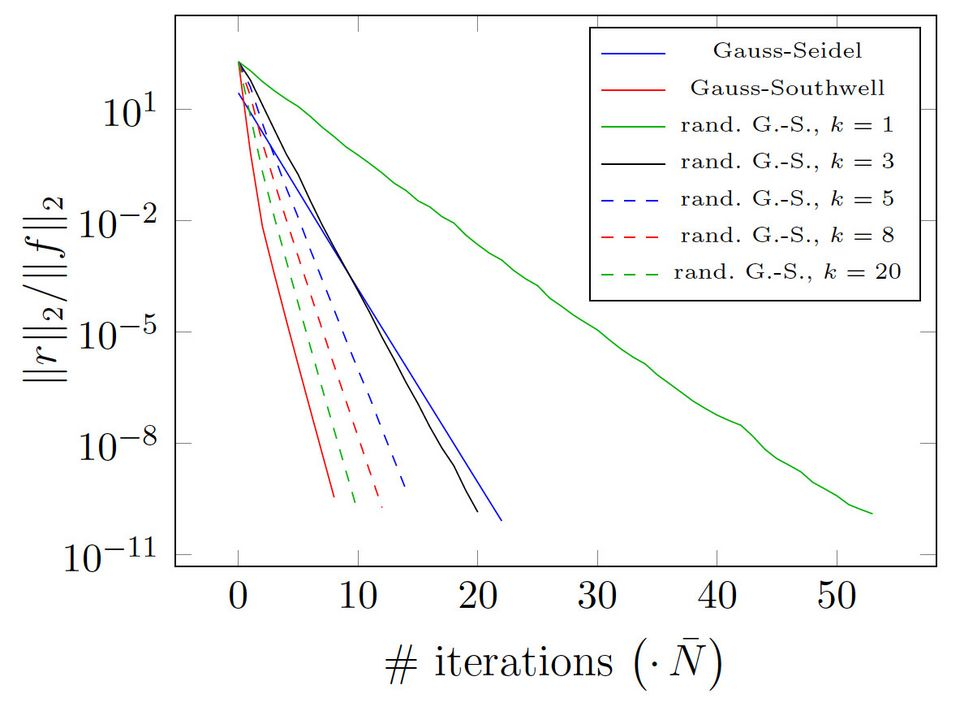## Statement

A major part of the compute time of many numerical methods is spend in the solution of large linear systems stemming back from discretizations. Large-scale problems require optimal-complexity linear solvers. Algebraic solution techniques such as algebraic multigrid (AMG) and hierarchical matrices allow to achieve optimal complexity without a too deep knowledge about the specifics of a discretization. Thereby they are of great interest for real-world applications.

However, the scalability of some of these methods on extreme-scale HPC clusters is in question. Future research should identify alternatives or improve existing techniques to remove scalability and potential resilience barriers. Moreover, algebraic solution techniques should be applied in a much broader context such as multi-level approximations.

## Exemplary Results

#### Algebraic multi-level frames# Algebraic multi-level frames can easily handle problems on complex geometries# Randomized and greedy-type solvers can be used together with the new algebraic multi-level frames

Hierarchical matrices

## Related Work

• H. Harbrecht and P. Zaspel. A scalable H-matrix approach for the solution of boundary integral equations on multi-GPU clusters. Preprint 2018-11, Fachbereich Mathematik, Universität Basel, Switzerland, 2018. Also available as arXiv:1806.11558.

• P. Zaspel. Algorithmic patterns for H matrices on many-core processors, accepted for publication in Journal of Scientific Computing, Springer, August 2018. Also available as Preprint 2017-12, Fachbereich Mathematik, Universität Basel, Switzerland, 2017 and as arXiv:1708.09707 preprint.

• H. Harbrecht, P. Zaspel. On the algebraic construction of sparse multilevel approximations of elliptic tensor product problems, accepted for publication in Journal of Scientific Computing, Springer, August 2018. Also available as Preprint 2018-01, Fachbereich Mathematik, Universität Basel, Switzerland, 2018 and as arXiv:1801.10532.

• P. Zaspel. Analysis and parallelization strategies for Ruge-Stüben AMG on many-core processors, Preprint 2017-06, Fachbereich Mathematik, Universität Basel, Switzerland, 2017.

• P. Zaspel. Subspace correction methods in algebraic multi-level frames. Linear Algebra and its Applications, Vol. 488(1),  Jan. 2016, pp. 505-521

• P. Zaspel. Parallel RBF Kernel-Based Stochastic Collocation for Large-Scale Random PDEs, PhD Thesis, Institute for Numerical Simulation, University of Bonn, Germany, Apr. 2015
###### Software
• hmglib: hierarchical matrices on graphic processing units (github)

• MPLA: multi-GPU parallel library for dense iterative matrix solvers (github)